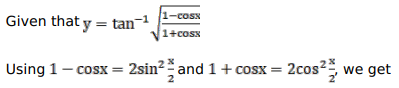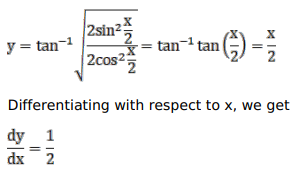# Solve this following

Question:

Mark $(\sqrt{)}$ against the correct answer in the following:

If $y=\tan ^{-1} \sqrt{\frac{1-\cos x}{1+\cos x}}$ then $\frac{d y}{d x}=$

A. $\frac{1}{2}$

B. $\frac{-1}{2}$

C. $\frac{1}{\left(1+x^{2}\right)}$

D. none of these

Solution: